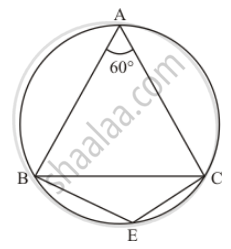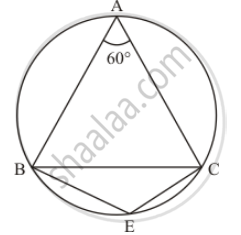Advertisement Remove all ads

# In the Given Figure, δAbc is an Equilateral Triangle. Find M∠Bec. - Mathematics

Short Note

In the given figure, ΔABC is an equilateral triangle. Find mBECAdvertisement Remove all ads

#### Solution

It is given that,   Δ ABC is an equilateral triangleWe have to find  m angle BEC

Since Δ ABC  is an equilateral triangle.

So angle A + angle B + angle C = 180°

And

angle A = angleB = angleC = 60°        …… (1)

Since, quadrilateral BACE  is a cyclic qualdrilateral

So ,  angle A + angleE = 180°       (Sum of opposite angles of cyclic quadrilateral is 180° .)

60° + angle E = 180°

angle E = 120°

Hence

m angle BEC = 120°

Is there an error in this question or solution?
Advertisement Remove all ads

#### APPEARS IN

RD Sharma Mathematics for Class 9
Chapter 15 Circles
Exercise 15.5 | Q 1 | Page 100
Advertisement Remove all ads
Advertisement Remove all ads
Share
Notifications

View all notifications

Forgot password?
Course